Study P6 Mathematics Angles - Geniebook# Finding Unknown Angles

In this article, we will learn how to find unknown angles. This is as per the course covered in Primary 6 Math level.

We will focus on the following topics:

• Finding unknown angles using properties of alternate angles
• Finding unknown angles using properties of geometric shapes such as triangles, squares, trapeziums, and circles
• Finding unknown angles when a paper is folded

## What is an Angle?

An angle is a figure formed by two rays that meet at a common endpoint. Angles can be divided into different categories depending on their measurement, for example, acute angle, obtuse angle, right angle, etc.

## Alternate Angles

What are alternate angles? When a straight line intersects two parallel lines, it is called a transverse line. When the transverse cuts across two parallel lines, a few angles are formed. The angles which are on the opposite side of the transverse are called alternate angles.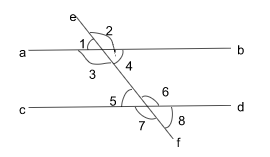### Alternate Angles

Angle 3 $$=$$ Angle 6

Angle 4 $$=$$ Angle 5

Let us study a few questions to further understand this concept and apply the property of alternate angles.

Question 1:

In the figure, not drawn to scale, $$AB$$ is parallel to $$CD$$. What is $$\angle BEF$$?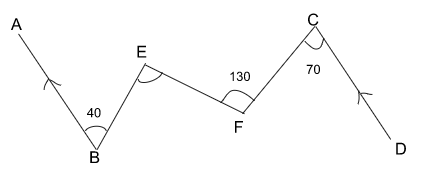Solution:

Let us draw lines at $$E$$ and $$F$$ parallel to $$AB$$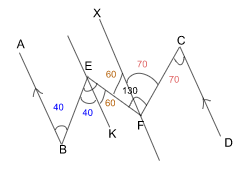From the above figure, we see that $$\angle BEF$$ is made from two sets of alternate angles

\begin{align*} \textstyle \angle DCF &= \angle XCF \\ &=70^\circ & \text{(alternate angles)} \\ \\ \angle XFE &= 130^\circ - 70^\circ \\ &=60^\circ\\\\ \angle XFE &= \angle FEK \\ &=60^\circ & \text{(alternate angles)} \\ \\ \angle ABE &= \angle BEK \\ &=40^\circ & \text{(alternate angles)} \\ \end{align*}

Hence,

\begin{align*} \textstyle \angle BEF &= \angle BEK +\angle FEK \\ &= 40^\circ + 60^\circ \\ &= 100^\circ\\ \therefore \angle BEF &= 100^\circ \end{align*}

$$\angle BEF = 100^\circ$$

## Finding Unknown Angles Using Geometric Shapes

In this section we will try to find unknown angles using geometric shapes such as squares, triangles, trapeziums etc.

Before we begin, let’s recall some properties about the common geometric shapes that we use.

### Square

• All the sides of a square are equal
• All the angles inside the square are $$90^\circ$$

### Equilateral Triangle

• All the sides of the equilateral triangle are equal
• All the angles inside the equilateral triangle are $$60^\circ$$

### Isosceles Triangle

• The two sides of an isosceles triangle are equal
• The two internal angles of the equal sides are equal

### Rectangle

• The opposite sides of the rectangle are equal
• The internal angles of the rectangle are 90º

Let’s put these concepts into practice and try to find unknown angles using these properties of the geometric shapes.

Question 2:

In the figure below, $$ABC$$ is a right angled triangle. $$EF$$ is parallel to $$GH$$. Find $$\angle BCH$$.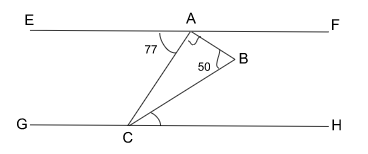Solution:

Since $$EF$$ is parallel to $$GH$$, $$\angle EAC = \angle ACH \qquad \text{(alternate angle)}$$

Triangle $$ABC$$ is a right angled triangle,

$$\angle A = 90^\circ, \angle B = 50^\circ$$

Sum of all the angles in a triangle is $$180^\circ$$, hence

\begin{align*} \textstyle \angle ACB &= 180^\circ - 90^\circ - 50^\circ \\ &=40^\circ \qquad\qquad\qquad\qquad \text{(sum of angles in triangle)}\\ \\ \text{Now, Since } \angle EAC &= \angle ACH \\ &=77^\circ \qquad\qquad\qquad\qquad \text{(alternate angles)}\\ \\ \angle BCH &= \angle ACH - \angle ACB \\ &=77^\circ - 40^\circ\\ &=37^\circ \end{align*}

$$\angle BCH = 37^\circ$$

Question 3:

In the figure, not drawn to scale, $$ABCD$$ is a square, $$DEC$$ is an equilateral triangle and $$CEFG$$ is a trapezium. $$EBF$$ is a straight line. Find $$\angle{CEB}$$.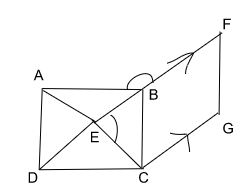Solution:

We know that $$ABCD$$ is a square. So $$AB = BC = CD = AD$$

We also know that $$\triangle{DEC}$$ is an equilateral triangle. We know that all the sides of an equilateral triangle are equal. Thus,

$$DE = CD = CE$$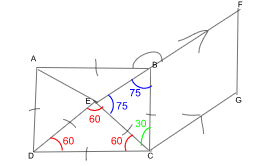So, from this we can see that $$\triangle{BCE}$$ is an Isosceles triangle.

Hence, $$CE = BC$$

In the $$\triangle{CBE}$$,

\begin{align}​​​ \angle{ECB} &= \angle{DCB} – \angle{DCE} \\ \angle{ECB} &= 90^\circ – 60^\circ\\ &= 30^\circ \end{align}

\begin{align}​​​ \textstyle \angle{ECB} &= 90^\circ – 60^\circ \\ &= 30^\circ \end{align}

Also, in an isosceles triangle, the two base angles are the same. Thus,

\begin{align}​​​ \textstyle \angle{CEB} &= \angle{CBE} \\ \angle{CEB} + \angle{CBE} &= 180^\circ – 30^\circ \\ &= 150^\circ ​​\end{align}

Therefore,

\begin{align} \textstyle \angle{CEB} &= 150^\circ /2 \\ &= 75^\circ ​​\end{align}

$$\angle{CEB} = 75^\circ$$

Question 4:

In the figure given below, not drawn to scale, $$ABCD$$ is a square and $$EDC$$ is an equilateral triangle. Find $$\angle{x}$$.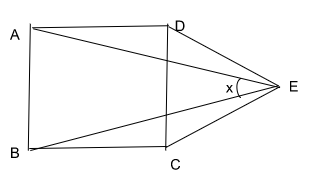Solution:

Here we know that$$ABCD$$ is a square.

So, $$AB = BC = CD = DA$$

Also, $$\triangle{CDE}$$  is an equilateral triangle.

Thus, $$CD = CE = DE$$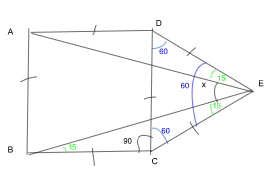Using the property of the Square, we know that all the angles are $$90º$$

Thus, $$∠BCD = 90º$$

Since, $$∆ CDE$$ is an equilateral triangle,

So, $$CD = BC = CE$$

Also, using the property of equilateral triangle, we know that all the angels are $$60º$$

Thus, $$∠DCE = 60º$$

From above we can infer that $$∆BCE$$ is an isosceles triangle

\begin{align}​​​ BC &= CE \\\\ ∠BCE &= ∠BCD + ∠DCE \\ &= 150º ​​\end{align}

Now, in the $$∆ BCE$$, we know that the sum of all the angles is $$180º$$

So,

\begin{align}\textstyle​​​ ∠BCE + ∠CBE + ∠CEB &= 180º \\ ∠CBE + ∠CEB &= 180º – 150º \\ &= 30º ​​\end{align}

Therefore,

\begin{align}​​​ ∠CBE &= ∠CEB \\ &= 15º ​​\end{align}

Similarly, $$\triangle{ADE}$$ is also an isosceles triangle

And $$∠DEA = 15º$$

But, it is given in the question that $$∆ CDE$$ is an equilateral triangle and all the angles are $$60º$$

Thus,

\begin{align}​​​ \textstyle ∠DEA + ∠AEB + ∠CEB &= 60º \\ ∠DEA + ∠CEB &= 15º + 15º \\ &= 30º ​​\end{align}

Since $$∆ CDE$$ is an equilateral triangle, $$∠DEC = 60º$$

\begin{align}​​​ ∠AEB &= 60º – 30º \\ ∠AEB &= 30º ​​\end{align}

$$∠AEB = 30º$$

## Finding Unknown Angles By Folding The Paper

When we fold a paper at a certain angle, and then when we unfold it, the edge of the paper forms a straight line. Do you know what are the properties of the angles in a straight line?

We know that the angles formed in a straight line add up to 180º. Now using this concept, try to find some unknown angles in a folded paper.

Question 5:

A rectangular piece of paper is folded as shown below. Find $$\angle a$$.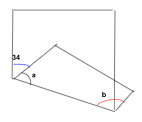Solution:

Let us see if we unfold this piece of paper, what will be the figure. We see from the figure below that the folded part is repeated.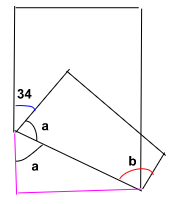Now we can see that if we unfold the paper, the edge of the paper forms a straight line.

We know that the sum of all the angles in a straight line is $$180º$$.

Thus,

\begin{align*} \textstyle \angle 34 + \angle a + \angle a &= 180^\circ \\ \angle a &= (180^\circ – 34^\circ) / 2 \\ \angle a &= 73^\circ \end{align*}

$$\angle a= 73^\circ$$

Question 6:

A rectangular piece of paper is folded as shown below. Find $$\angle b$$.Solution:

We have already found that $$\angle a= 73^\circ$$

When we unfold the paper, we know that the paper is a rectangle and so the edges of the paper are parallel lines.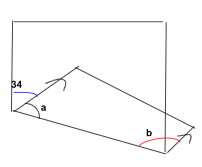Now we can see that $$\angle a$$ and $$\angle b$$ are the angles between two parallel line.

According to the property of the parallel lines, we know that the sum of angles between two parallel lines is $$180^\circ$$.

Therefore,

\begin{align}​​​ \textstyle \angle{a} + \angle{b} &= 180^\circ \\ \angle{a} &= 73^\circ \\ \angle{b} &= 107^\circ ​​\end{align}

$$\angle b= 107^\circ$$

Question 7:

In the figure, not drawn to scale, a rectangular piece of paper was folded along $$EF$$ as shown below. Given that $$\angle AED= 124^\circ$$, find $$\angle{AFB}$$.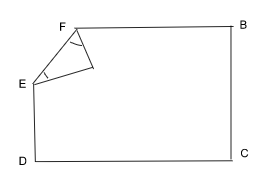Solution:

Let us unfold the paper along $$EF$$ and see the figure.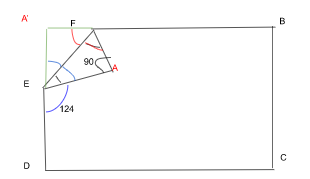After unfolding the paper at $$EF$$, we see that it is rectangular paper.

Thus the angle at A is $$90^\circ$$.

We see that $$AED$$ is a straight line.

\begin{align}​​​ \textstyle \angle{A'EF} + \angle{FEA} + \angle{AED} &= 180^\circ ​​\end{align}

Once the paper fold is unfolded, the folded part is repeated.

Therefore,

\begin{align}​​​ \textstyle \angle{A'EF} &= \angle{FEA} \\ &= 180^\circ -124^\circ \\ \\ \angle{FEA} &= 28^\circ ​​\end{align}

Now the $$\triangle{AEF}$$ is a right angled triangle.

Therefore,

\begin{align}​​​ \textstyle \angle{FEA} + \angle{AFE} &= 90^\circ \\ \angle{AFE} &= 62^\circ ​​\end{align}

Now, $$A′FB$$ is a straight line. So the sum of the angles on it is $$180^\circ$$.

Thus,

\begin{align*}​​​ \textstyle \angle{A′FE} + \angle{AFE} + \angle{AFB} = 180^\circ ​​\end{align*}

Since,

\begin{align*}​​​ \textstyle \angle{A′FE} &= \angle{AFE} \\ &= 62^\circ \\ \\ \angle{AFB} &= 56^\circ ​​\end{align*}

$$\angle{AFB} = 56^\circ$$

Question 8:

In the figure, not drawn to scale, a rectangular piece of paper is folded along $$AD$$ as shown. Given that $$EFBC$$ is a straight line, find $$\angle{EDF}$$ and $$\angle{DFE} ,\,\angle{DFC}$$.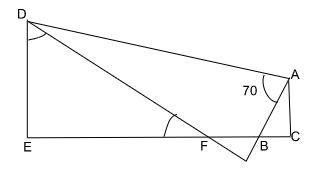Solution:

When we unfold the paper at $$AD$$, then the folded part is repeated.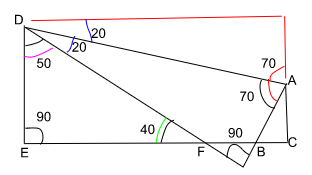From the above figure, we can see that the folded angles are repeated once they are unfolded.

Then using the properties of the right–angled triangle, we can find out the respective angles.

We see that

\begin{align*}​​​ \textstyle \angle{EDF} &= 50^\circ \\ \angle{DFE} &= 40^\circ ​​\end{align*}

Since $$EFBC$$ is a straight line, then the sum of the angles on it is $$180^\circ$$.

\begin{align*}​​​ \textstyle \angle{DEF} &= 140^\circ ​​\end{align*}

\begin{align*}​​​ \textstyle \angle{EDF} = 50^\circ, \;\angle{DFE} = 40^\circ \text{ and } \;\angle{DEF} = 140^\circ ​​\end{align*}

## Conclusion

• We learned how to find unknown angles using the properties of alternate angles in parallel lines.
• We also learned the properties of some of the commonly used geometric figures like squares, triangles, trapezium, rectangle,s etc. We learned how to find unknown angles using these properties.
• We understood the technique of finding the unknown angles using the folded paper. We learned that once we unfold the paper, the folded part is repeated, and thus the angles in the folded part get repeated too.

Hope this article was helpful to you in trying to understand various ways of finding unknown angles. Do revisit the above topics again to get a better grip on these concepts. Practice makes perfect! Keep going!

 Continue Learning Algebra Distance, Speed and Time Volume of Cubes and Cuboid Fundamentals Of Pie Chart Finding Unknown Angles Number Patterns: Grouping & Common Difference Fractions Of Remainder Fractions - Division Ratio Repeated Identity: Ratio StrategiesPrimaryPrimary 1Primary 2Primary 3Primary 4Primary 5Primary 6EnglishMaths
Algebra
Distance, Speed and Time
Volume of Cubes and Cuboid
Fundamentals Of Pie Chart
Finding Unknown Angles
Number Patterns: Grouping & Common Difference
Fractions Of Remainder
Fractions - Division
Ratio
Repeated Identity: Ratio StrategiesScienceSecondarySecondary 1Secondary 2Secondary 3Secondary 4